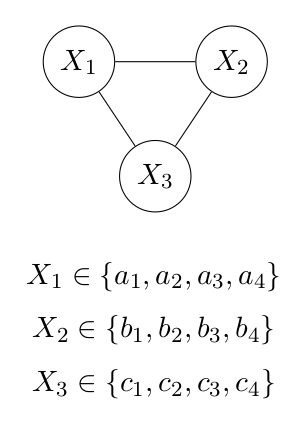# Pseudolikelihood in Graphical Models

## Introduction

Because I did not use pseudolikelihood quite frequently, I often forget what it is and how to calculate it. The introductory materials from Wikipedia and other resources are always vague and confusing to beginners. So I decided to write a blog post on pseudolikelihood in detail in case in the future I forget it again.

## Problem Definition

We usually have to calculate, given a graphical model (directed or undirected), what the probability of each possible state is. Concretely, we have the following undirected graphical model. We would like to know $\text{Pr}(X_1 = a_2, X_2 = b_3, X_3 = c_2)$.$X_1, X_2, X_3$ can take 4 different values, respectively. We also ignore the value of the edges. The score of the state $S(X_1, X_2, X_3)$ in the graph could be calculated using $f(X_1, X_2, X_3)$. To calculate $\text{Pr}(X_1 = a_2, X_2 = b_3, X_3 = c_3)$, we will usually have to first calculate the scores for all the possible states in the graph and apply normalization functions to turn the scores to probabilities. Usually the normalization function could be softmax function.

$$Z = \sum_{1}^{4} \sum_{1}^{4} \sum_{1}^{4} e^{f(X_1 = a_i, X_2 = b_i, X_3 = c_i)}$$

$$\text{Pr}(X_1 = a_i, X_2 = b_i, X_3 = c_i) = e^{f(X_1 = a_i, X_2 = b_i, X_3 = c_i)}/Z$$

Therefore,

$$\text{Pr}(X_1 = a_2, X_2 = b_3, X_3 = c_2) = e^{f(X_1 = a_2, X_2 = b_3, X_3 = c_2)}/Z$$

More generally, if there are $n$ nodes in the graph and each node could choose from $k$ different values. The complexity of calculating $\text{Pr}(X)$ will be $O(k^n)$. When the graphical model becomes larger and more complex, calculating $\text{Pr}(X)$ becomes intractable.

Without applying softmax function globally, the probability of the state $S(X_1, X_2, X_3)$ could also be calculated from the “chain-rule” of conditional probabilities:

\begin{align} \text{Pr}(X_1 = a_2, X_2 = b_3, X_3 = c_2) & = \text{Pr}(X_3 = c_3 | X_2 = b_3, X_1 = a_2) \\ & \times \text{Pr}(X_2 = b_3 | X_1 = a_2) \\ & \times \text{Pr}(X_1 = a_2) \end{align}

However, to calculate the conditional probabilities on the right side, it is still required to calculate the probabilies of all the states, which still takes $O(k^n)$.

## Pseudolikelihood Approximation

$$\text{Pr}(X = x) = \prod_{i=1}^{n} \text{Pr}(X_i = x_i | x_{-i})$$

In our case,

\begin{align} \text{Pr}(X_1 = a_2, X_2 = b_3, X_3 = c_2) & = \text{Pr}(X_1 = a_2 | X_2 = b_3, X_3 = c_2) \\ & \times \text{Pr}(X_2 = b_3 | X_1 = a_2, X_3 = c_2) \\ & \times \text{Pr}(X_3 = c_3 | X_1 = a_2, X_2 = b_2) \end{align}

We first calculate $\text{Pr}(X_1 = a_2 | X_2 = b_3, X_3 = c_2)$.

Given $X_2 = b_3, X_3 = c_2$, $X_1$ has four possible values $a_1, a_2, a_3, a_4$. We can therefore calculate the socres for the four possible states $S(X_1 = a_1, X_2 = b_3, X_3 = c_2)$, $S(X_1 = a_2, X_2 = b_3, X_3 = c_2)$, $S(X_1 = a_3, X_2 = b_3, X_3 = c_2)$, $S(X_1 = a_4, X_2 = b_3, X_3 = c_2)$.

$$Z’ = \sum_{1}^{4} e^{f(X_1 = a_i, X_2 = b_3, X_3 = c_2)}$$

Apply softmax function to calculate $\text{Pr}(X_1 = a_2 | X_2 = b_3, X_3 = c_2)$

$$\text{Pr}(X_1 = a_2 | X_2 = b_3, X_3 = c_2) = e^{f(X_1 = a_2, X_2 = b_3, X_3 = c_2)}/Z’$$

Similarly, we can calculate $\text{Pr}(X_2 = b_3 | X_1 = a_2, X_3 = c_2)$ and $\text{Pr}(X_3 = c_3 | X_1 = a_2, X_2 = b_2)$

Once the conditional probabilities have all been calculated, we could calculate the pseudolikelihood of state $\text{Pr}(X_1 = a_2, X_2 = b_3, X_3 = c_2)$ by multiplying the three conditional probabilities together.

More generally, if there are $n$ nodes in the graph and each node could choose from $k$ different values. The complexity of calculating the pseudolikelihood $\text{Pr}(X)$ will be $O(kn)$, which is much more efficient than calculating the true likelihood.

## Conclusion

Pseudolikelihood makes the calculation of the probability of the state from “intractable” to “tractable”, which accelerates our computation of the graphical models.

Pseudolikelihood in Graphical Models

https://leimao.github.io/blog/Pseudolikelihood/

Lei Mao

02-16-2018

02-16-2018